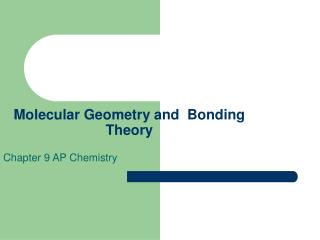# Molecular Geometry and Bonding Theory - PowerPoint PPT PresentationDownload PresentationMolecular Geometry and Bonding Theory

Molecular Geometry and Bonding TheoryDownload Presentation## Molecular Geometry and Bonding Theory

- - - - - - - - - - - - - - - - - - - - - - - - - - - E N D - - - - - - - - - - - - - - - - - - - - - - - - - - -
##### Presentation Transcript

1. Molecular Geometry and Bonding Theory Chapter 9 AP Chemistry

2. Molecular Geometry • Molecular Geometry- general shape of a molecule as determined by the relative position of the nuclei. • The geometry and size of a molecule helps to determine it’s chemical behavior. • VSEPR- Valence-Shell-Electron-Pair-Repulsion model- predicts geometry based upon e’s around the central atom.

3. Electrons are kept as far away from one another as possible – minimizing e pair repulsions Electron pairs are considered as being bonding or non-bonding (lone pairs) A multiple bond counts as a single bonding pair Electron pair geometry is described by the regions of e’s around the central atom Molecular geometry is a consequence of electron pair geometry. Principles of the VSEPR theory

4. Predicting Structures VSEPR • Derive the Lewis Structure to the form AXmEn • A = central atom • X = atoms bonded to the central atom • E = lone pairs of e’s on the central atom • M = # of bonded atoms • N = # of lone pairs

5. Example Electron and Molecular Geometry Linear Bond angles 180 Sp Hybrid Non-polar Electron Geometry Linear

6. More Examples AX2

7. Electron Geom. Trigonal Planar • Molecular Geom. Trigonal Planar bond angles exactly 120 non-polar Bent bond angles aprox 120 polar molecule • Sp2 hybridized

8. Electron Geom.Trigonal Planar • Molecular Geometry: AX3 (3BP or 2BP + 1LP) ex. 3bp NO3-                                 <>

9. Examples Cont. 3bp • BF3 <>                    <>

10. Examples Cont. 2bp + 1 lp • Ex O3 (ozone)

11. Electron Geom. Tetrahedral • Molecular Geom. Tetrahedral bond angles exactly 109.5 non-polar molecule, Trigonal pyramidal bond angles aprox. 109.5 polar molecule, Bent bond angles aprox. 109.5 polar molecule • Sp3 hybridized

12. Molecular Geometry: AX4 [4BP or (3BP + 1LP) or (2BP + 2LP)] • Electron Geometry: AX4Tetrahedral

13. Example • BrO3F, Perbromyl fluoride

14. Molecular Geometry: AX3E1Trigonal Pyramidal

15. Example • NF3, Nitrogen trifluoride

16. Molecular Geometry: AX2E2Bent/Angular

17. Example ClOF, Chlorosyl fluoride • H2O, Water,

18. Electron Geom. Trigonal Bypyramidal • Mol. Geom. Trigonal Bypyramidal – bond angles ax. exactly 108 deg. eq. exactly 120 deg. Non-polar, See-Saw – bond angles ax. aprox. 108 deg. eq. aprox. 120 deg. Polar, T-shaped - bond angles ax. aprox. 108 deg. No –eq, Linear – bond angle exactly 180 non-polar. • All sp3d hybridized

19. Mol geo. Trigonal bipramidal • AX5 [5BP or (4BP + 1 LP) or (3BP + 2LP) or (2BP + 3LP)] • 5bp

20. Example • PCl5(gas phase), Phosphorous pentachloride

21. Molecular Geometry:AX4E1See-saw • Example IF2O2-

22. Molecular Geometry:AX3E2T-structure • Example ClF3

23. Molecular Geometry:AX2E3Linear • Examole XeF2

24. Electron Geometry Octahedrial • Mol geo. Octahedral – bond angle exactly 90 - non-polar, square pyramidal – bond angle aprox 90- polar, square planar- bond angle exactly 90 non-polar • Sp3d2 hybridized

25. Molecular Geometry: AX6Octahedral • Example SF6

26. Molecular Geometry: AX4E1Pyramidal Planar • Example XeOF4

27. Molecular Geometry: AX4E2Square Planar • Example XeF4

28. Bond Angles • Non-bonding pairs of e’s take up more space (att. by one nucli) than bonded e pairs • Double and triple bonds take up more space than single bonds (more e’s) • Volume occupied lone pairs > triple bonds > double bonds > single bonds

29. Forces • Non-bonding pairs exert repulsive forces on adjacent e pairs and compress angles • Multiple bonds also exert repulsive forces and compress angles